# Ion-Slip Effects on Bingham Fluid Flowing Through an Oscillatory Porous Plate with Suction

Ion-Slip Effects on Bingham Fluid Flowing Through an Oscillatory Porous Plate with Suction

Md. Tusher Mollah Henrik K. Rasmussen Saykat Poddar Muhammad Minarul Islam Mehetaj Parvine Md. Mahmud Alam Giulio Lorenzini*

Department of Mechanical Engineering, Technical University of Denmark, 2800 Kgs. Lyngby, Denmark

Department of Mathematics, Bangabandhu Sheikh Mujibur Rahman Science and Technology University, Gopalganj 8100, Bangladesh

Mathematics Department, Patuakhali Science and Technology University, Patuakhali 8602, Bangladesh

Mathematics Discipline, Khulna University, Khulna 9208, Bangladesh

Department of Engineering and Architecture, University of Parma, Parco Area delle Scienze 181/A, Parma 43124, Italy

Corresponding Author Email:
giulio.lorenzini@unipr.it
Page:
673-681
|
DOI:
https://doi.org/10.18280/mmep.080501
3 March 2021
|
Accepted:
1 September 2021
|
Published:
31 October 2021
| Citation

OPEN ACCESS

Abstract:

This paper investigates the remarkable effects for introducing the Ion-slip characteristics in the magnetohydrodynamic phenomena on the drift of Bingham fluid when flowing through the inner territory of two porous parallel plates in correspondence with the suction case. The lower plate (L-plate) is as steady while the upper one (U-plate) is oscillatory, which oscillates in its own plane at time t>0. A magnetic field, which is uniform, is affixed perpendicular to the plates. The U-plate temperature oscillates while the L-plate temperature is constant. Numerical performance is presented by the MATLAB R2015a simulation tool with the explicit Finite difference Method (FDM) algorithm. To ensure the preciseness and convergence of the solutions, careful attention has been given on the criteria of stability and convergence of the FDM schemes. As an outcome, the converged solution is obtained for Pr ≥ 0.066, βi ≥ 2, Ha ≤ 20, k0 ≤ 8, S ≥ −10 and Re ≥ −0.017 with the arbitrary choice of βe= 0.10 and Ec= 0.01. The mesh sensibility test gives the competent mesh space at (m,n)=(60,60). The time sensibility test ensures that the solutions at dimensionless time, τ=2.0 will be steady-state. The exactitude of the current study is obtained by comparing with the published results. Finally, the physical influences of several governing parameters, including Ion-slip on the fluid property like velocity, local shear stress, temperature and Nusselt number are discussed and decorated graphically.

Keywords:

MHD, heat transfer, Bingham fluid, suction, oscillatory porous plate, finite difference method

1. Introduction

The Magnetohydrodynamic (MHD) fluid passing a porous medium has attracted much attention in various scientific and engineering applications in industry. Ample applications of the flows via medium of porosity are found for petroleum processing problems, filtration processes and oil purification processes, biological mechanisms as well as polymer decomplex industries. Numerous investigations have been considered on multifarious MHD non-Newtonian flow configuration such as Human blood, slurries, molten plastics, tomato sauce, honey, soup, mud, lava, painting oil, cement, sludge, grease, chocolate etc. passing through porous oscillating plate.

The foremost approach to establish an exact solution of the Navier-Stokes’ equation over the oscillating plate has been studied in Ref.  which is concerned with the flow past a horizontal oscillatory plate. In the works , the oscillatory MHD flow flowing through a flat plate with Hall impacts is considered. The free convection-radiation for the MHD oscillating flow through a medium of porous material which is circumscribed by plane surface of vertical orientation has been investigated (see Ref. ). According to Chaudhary and Jain , the free convection flow flowing over an oscillatory and porous flat vertical plate with the mixed heat and mass exchange effects is studied. The study of unsteady MHD Hartmann–Couette flow through a Darcian channel be with Ion-slip, Hall current including Joule heating effects has been inquired by Bég et al. . In Ref. , the MHD flow passing over an oscillating porous plate bewith Soret impact and thermal radiation are investigated. The solution by FEM for MHD flow flowing through time depending permeable medium of porous material correspondence with the suction velocity of oscillatory nature has been carried out by Rao e al. . Manna et al.  studied the impressions of radiation and oscillatory heat flux on the unsteady MHD viscous incompressible flow past an oscillating porous plate bewith the consideration of free convection. The study on a three-dimensional oscillatory flow passing along a porous plate bewith Dufour and Soret effects has been assumed by Ahmed et al. . In a rotating system, the study of MHD flow including free convection also mass transfer while flowing through an oscillatory porous plate along with heat source, Ion-slip, and Hall currents have been investigated (see Ref. ). The unsteady MHD viscous incompressible free convective flow for non- Newtonian Casson fluid flowing over an oscillating porous plate is studied by Khalid et al. . In a Rotating Porous Medium, the study of MHD fluid flowing through an oscillatory porous plate bewith Ion-slip and Hall currents has been considered in works . The impression silver nanoparticles on the flow of non-Newtonian Jeffrey fluid through an oscillating porous vertical plate with the magnetic field has been investigated (see the Ref. ). The MHD radiating and chemically reacting flow criteria for the Casson fluid through oscillatory vertical plate inserted in the porous medium are considered . The unsteady MHD flow of viscoelastic fluid under the presence of heat source along with an infinitely long plate with oscillation has been studied by Sharmin and Alam . The Impact of Ion-slip and Hall currents for the unsteady flow of MHD free convective incompressible rotating fluid past an oscillatory vertical plate has been considered by Singh et al. . The Soret and Hall impact on the study of unsteady MHD flow passing over a porous plate with changing suction criteria through a varying permeable medium are investigated . For Bingham fluid, the Hall and Ion-slip effects through parallel plates [18, 19], and the EMHD flow through parallel Riga plates [20, 21] have been discussed. However, the Bingham fluid flow through an oscillatory channel with different physical aspects is yet to understand.

The objective is to make a thorough understanding of the Ion-slip responses on the flow of Bingham fluid through oscillatory porous parallel plates with the consideration of suction velocity. First, the use of a compatible scaling group of transformations has been employed to obtain the dimensionless governing non-linear PDEs. Then employment of explicit FDM leads to obtain the solution of the dimensionless PDEs. Finally, the resulting features have been discussed with validation and expressed graphically.

2. Mathematical Formulation

The analysis deals with the flow of incompressible, laminar Bingham fluid under the convenient choice of two infinitely long plates of horizontal orientation which are placed at the planes (Figure 1) defined as y=±h while the lengthening ranges are from x=0 to $\infty$ and from z=0 to $\infty$. A uniform suction is considered for the fluid motion. Before the immediate starting of unsteadiness i.e. at t=0, the fluid and the U-plate are presumed at static state with temperature T1. Then while unsteadiness happens i.e. at t>0, the U-plate commences own plane oscillation in a specified direction with the reference velocity ${{U}_{0}}\cos \omega t$, additionally, the temperature of the plate is promoted to T2. At the U-plate a temperature of periodic nature is instated in addition to the fixed mean temperature. The L-plate temperature is supposed to be unchangeable with T1, where T2> T1.

A constant pressure gradient $\frac{dp}{dx}$is employed in the X-direction within the flow zone along with a negligible assumption of body force. Applied uniform magnetic field B0, is deemed in the Y-direction, whereas the existence of small magnetic Reynolds number discloses an inconsiderable magnetic induction. A Z-element is expected to occur in the velocity for the causation of Hall current. For the uniform suction, $\frac{\partial \tilde{u}}{\partial x}=0$therefore, continuity equation $\frac{\partial \tilde{u}}{\partial x}+\frac{\partial \tilde{v}}{\partial y}=0$ forms $\frac{\partial \tilde{v}}{\partial y}=0$, i.e. $\tilde{v}=-{{v}_{0}}$. The vector form of fluid velocity is given as $\mathbf{q}=\tilde{u}\left( y,t \right)\mathbf{i}-{{v}_{0}}\mathbf{j}+\tilde{w}\left( y,t \right)\mathbf{k}.$

## 1.pngFigure 1. The physical configuration of the problem

Within the outlines of the above discretions, the governing equations related to Ion-slip responses on the flow of Bingham fluid through oscillatory porous parallel plates with the controlling of suction velocity under the boundary-layer approximations are ([22-25]):

Continuity Equation:

$\frac{\partial \tilde{v}}{\partial y}=0$     (1)

Momentum Equations:

\begin{align} & \frac{\partial \tilde{u}}{\partial t}-{{v}_{0}}\frac{\partial \tilde{u}}{\partial y}=-\frac{1}{\rho }\frac{dp}{dx}+\frac{1}{\rho }\frac{\partial }{\partial y}\left( \tilde{\mu }\frac{\partial \tilde{u}}{\partial y} \right) \\ & \text{ }-\frac{1}{\rho }\frac{{{\sigma }_{\text{e}}}B_{_{0}}^{2}}{\alpha _{\text{e}}^{2}+\beta _{\text{e}}^{2}}\left[ {{\alpha }_{e}}\tilde{u}+{{\beta }_{e}}\tilde{w} \right]-\frac{\upsilon }{k'}\tilde{u} \\\end{align}     (2)

$\frac{\partial \tilde{w}}{\partial t}-{{v}_{0}}\frac{\partial \tilde{w}}{\partial y}=\frac{1}{\rho }\frac{\partial }{\partial y}\left( \tilde{\mu }\frac{\partial \tilde{w}}{\partial y} \right)-\frac{1}{\rho }\frac{{{\sigma }_{\text{e}}}B_{_{0}}^{2}}{\alpha _{\text{e}}^{2}+\beta _{\text{e}}^{2}}\left[ {{\alpha }_{e}}\tilde{w}-{{\beta }_{e}}\tilde{u} \right]-\frac{\upsilon }{k'}\tilde{w}$      (3)

Energy Equation:

\begin{align} & \frac{\partial \tilde{T}}{\partial t}-{{v}_{0}}\frac{\partial \tilde{T}}{\partial y}=\frac{\kappa }{{{c}_{p}}\rho }\left( \frac{{{\partial }^{2}}\tilde{T}}{\partial {{y}^{2}}} \right)+\frac{{\tilde{\mu }}}{\rho {{c}_{p}}}\left[ {{\left( \frac{\partial \tilde{u}}{\partial y} \right)}^{2}}+{{\left( \frac{\partial \tilde{w}}{\partial y} \right)}^{2}} \right] \\ & \text{ }+\frac{1}{\rho {{c}_{p}}}\frac{\sigma _{\text{e}}^{\text{2}}B_{_{0}}^{2}}{\alpha _{\text{e}}^{2}+\beta _{\text{e}}^{2}}\left( {{{\tilde{u}}}^{2}}+{{{\tilde{w}}}^{2}} \right) \\\end{align}      (4)

where,

$\tilde{\mu }=K+\frac{{{\tau }_{0}}}{\sqrt{{{\left( \frac{\partial \tilde{u}}{\partial y} \right)}^{2}}+{{\left( \frac{\partial \tilde{w}}{\partial y} \right)}^{2}}}}$      (5)

The criteria for the initial and boundary settings are:

$t\le 0,\,\,\,\,\,\tilde{u}=0,\tilde{w}=0,\tilde{T}={{T}_{1}}$ everywhere     (6)

\begin{align} & \,\,\,\,\,\,\,\,\,\,\,\,\,\,\,\tilde{u}\to 0,\tilde{w}=0,\tilde{T}={{T}_{1}}\,\,\,\,\text{at}\,\,x\text{=0} \\ & t>0,\text{ }\tilde{u}=0,\tilde{w}=0,\tilde{T}={{T}_{1}}\,\,\,\,\text{at}\,\,y=-h \\ & \,\,\,\,\,\,\,\,\,\,\,\,\,\,\,\left. \begin{matrix} \tilde{u}={{U}_{0}}\,\cos \omega t,\,\tilde{w}=0,\ \ \ \ \\ \tilde{T}={{T}_{2}}+\left( {{T}_{2}}-{{T}_{1}} \right)\cos \omega t\, \\\end{matrix} \right\}\ \text{at}\,\,\,y=h \\\end{align}     (7)

The following use of compatible scaling group of transforming variables have been employed to make the governing equations (1) to (5) dimensionless as the solution is based on explicit FDM concerning the conditions (6) and (7):

\begin{align} & X=\frac{x}{h},Y=\frac{y}{h},U=\frac{{\tilde{u}}}{{{U}_{0}}},W=\frac{{\tilde{w}}}{{{U}_{0}}},P=\frac{p}{\rho U_{0}^{2}}, \\ & \tau =\frac{t{{U}_{0}}}{h},\theta =\frac{\tilde{T}-{{T}_{1}}}{{{T}_{2}}-{{T}_{1}}},\bar{\mu }=\frac{{\tilde{\mu }}}{K},\eta =\frac{\omega h}{{{U}_{0}}} \\\end{align}     (8)

The above process possesses expected non-linear PDEs as follows:

\begin{align} & \frac{\partial U}{\partial \tau }-\frac{S}{{{R}_{e}}}\frac{\partial U}{\partial Y}=-\frac{dP}{dX} \\ & \text{ +}\frac{1}{{{R}_{e}}}\left[ \frac{\partial }{\partial Y}\left( \bar{\mu }\frac{\partial U}{\partial Y} \right)-\frac{H_{a}^{2}}{\alpha _{\text{e}}^{2}+\beta _{\text{e}}^{2}}\left( {{\alpha }_{e}}U+{{\beta }_{e}}W \right) \right]-{{k}_{0}}U \\\end{align}      (9)

\begin{align} & \frac{\partial W}{\partial \tau }-\frac{S}{{{R}_{e}}}\frac{\partial W}{\partial Y}=\frac{1}{{{R}_{e}}}\left[ \frac{\partial }{\partial Y}\left( \bar{\mu }\frac{\partial W}{\partial Y} \right)-\frac{H_{a}^{2}}{\alpha _{\text{e}}^{2}+\beta _{\text{e}}^{2}}\left( {{\alpha }_{e}}W-{{\beta }_{e}}U \right) \right] \\ & \text{ }-{{k}_{0}}W \\\end{align}     (10)

\begin{align} & \frac{\partial \theta }{\partial \tau }-\frac{S}{{{R}_{e}}}\frac{\partial \theta }{\partial Y}=\frac{1}{{{P}_{r}}}\frac{{{\partial }^{2}}\theta }{\partial {{Y}^{2}}}+{{E}_{c}}\bar{\mu }\left[ {{\left( \frac{\partial U}{\partial Y} \right)}^{2}}+{{\left( \frac{\partial W}{\partial Y} \right)}^{2}} \right] \\ & \text{ }+\frac{{{E}_{c}}H_{a}^{2}}{\alpha _{\text{e}}^{2}+\beta _{\text{e}}^{2}}\left[ {{U}^{2}}+{{W}^{2}} \right] \\\end{align}      (11)

$\bar{\mu }=1+\frac{{{\tau }_{D}}}{\sqrt{{{\left( \frac{\partial U}{\partial Y} \right)}^{2}}+{{\left( \frac{\partial W}{\partial Y} \right)}^{2}}}}$     (12)

The corresponding dimensionless criteria for the initial and boundary settings are:

$\tau \le 0,\,\,\,\,\,U=0,W=0,\theta =0$ everywhere       (13)

\begin{align} & \,\,\,\,\,\,\,\,\,\,\,\,\,\,\,\,\,U\to 0,W=0,\theta =0\,\,\,\,\text{at}\,\,X\text{=0} \\ & \tau >0,\text{ }U=0,W=0,\theta =0\,\,\,\,\text{at}\,\,Y=-1 \\ & \,\,\,\,\,\,\,\,\,\,\,\,\,\,\,\,\,U=\cos \eta \tau ,W=0,\theta =1+\,\cos \eta \tau \,\,\,\text{at}\,\,\,Y=1 \\\end{align}      (14)

The dimensionless quantities are: ${{\tau }_{D}}=\frac{{{\tau }_{0}}h}{K{{U}_{0}}}$ (Bingham number or dimensionless yield stress), ${{R}_{e}}=\frac{\rho {{U}_{0}}h}{K}$ (Reynolds number), ${{P}_{r}}=\frac{\rho {{c}_{p}}{{U}_{0}}h}{k}$ (Prandtl number), ${{E}_{C}}=\frac{{{U}_{0}}K}{\rho {{c}_{p}}h({{T}_{2}}-{{T}_{1}})}$ (Eckert number), $H{{a}^{2}}=\frac{\sigma B_{0}^{2}{{h}^{2}}}{K}$ (Hartmann number squared), $S=\frac{\rho {{V}_{0}}h}{K}$ (Suction Parameter) and ${{k}_{0}}=\frac{{{\upsilon }^{2}}}{k{{U}_{0}}^{2}}$ (Permeability of porous medium).

3. Shear Stress and Nusselt Number

The nature of fluid velocity vicinity to both the L-plate (static plate) and U-plate (oscillatory plate) create the scope for the calculations of the shear stresses. In X-direction the local shear stress for L-plate is:

${{\tau }_{w1}}\equiv {{\left[ \bar{\mu }\sqrt{{{\left( \frac{\partial U}{\partial Y} \right)}^{2}}+{{\left( \frac{\partial W}{\partial Y} \right)}^{2}}} \right]}_{Y=-1}}$,      (15)

and for U-plate is:

${{\tau }_{w2}}\equiv {{\left[ \bar{\mu }\sqrt{{{\left( \frac{\partial U}{\partial Y} \right)}^{2}}+{{\left( \frac{\partial W}{\partial Y} \right)}^{2}}} \right]}_{Y=1}}$.      (16)

Also, the temperature vicinity to both the L-plate (static plate) and U-plate (oscillatory plate) create the scope for the calculations of the Nusselt numbers. So, the mathematical representations of the local Nusselt number in X-direction for L-plate is:

${{N}_{u1}}\equiv \frac{{{\left( \frac{\partial T}{\partial Y} \right)}_{Y=-1}}}{-{{T}_{m}}}$,       (17)

and for U-plate is:

${{N}_{u2}}\equiv \frac{{{\left( \frac{\partial T}{\partial Y} \right)}_{Y=1}}}{-\left( {{T}_{m}}-1 \right)}$,      (18)

where, ${{T}_{m}}=\frac{\int\limits_{-1}^{1}{U\theta dY}}{\int\limits_{-1}^{1}{UdY}}$.

4. Calculation Technique

The explicit FDM has discretized the mathematical equations (9) to (12) of the physical problem, and consequently, the equations disclose some numerically discretized equations which are then solved via MATLAB under the specified boundary conditions. This discretization technique is conducted for flow space through backward type differencing regarding first-order differential term and through central type differencing regarding the higher-order differential term. The time-domain has been severed in possible small quantities where the last time value is used to compute the next time value for all the profiles. The territory covered by the boundary layer is allocated with the help of several grid lines to the normal direction of $Y$-axis (see Figure 2).

The maximum length of the limiting layer is supposed to be ${{Y}_{\max }}\left( =2 \right)$ which corresponds to $Y\to \infty$ i.e. the range of Y starts from 0 and ends to 2. Along the Y-direction, n=60 is supposed to be the number of grid spacing. This supposition discloses the uniform mesh size as:

$\Delta Y=0.0\text{33}\,\left( 0\le y\le 2 \right)$;

with the possible smaller time-step, $\Delta \tau =0.0001$. Some successful studies within a similar calculation framework can be found in Refs. [26-31].

## 2.pngFigure 2. The finite difference grid space

5. Stability and Convergence Analysis

The stability interpretation of the FDM is important since an explicit scheme is being used. To obtain the converged solution, following Fourier expansion for $U$,$U'$, $W$, $W'$ and $\theta$, $\theta '$ are defined in terms of $\exp \left( ibY \right)$ at arbitrary time τ=0:

$U,U'\,=\,\left( \chi \left( \tau \right),\chi '\left( \tau \right) \right)\exp \left( ibY \right)$

$W,W'\,=\,\left( \xi \left( \tau \right),\xi '\left( \tau \right) \right)\exp \left( ibY \right)$

$\theta ,\theta '\,=\,\left( \zeta \left( \tau \right),\zeta '\left( \tau \right) \right)\exp \left( ibY \right)$

Using the above expressions to Eqns. (15) to (17), a matrix notation is obtained with a constant matrix, called the amplification matrix. For stability, each eigenvalue of that constant matrix must not exceed unity in modules. Hence, the stability with convergence criteria can finally be expressed as follows:

$\frac{\Delta \tau }{2{{R}_{e}}}\frac{H_{a}^{2}}{\alpha _{\text{e}}^{2}+\beta _{\text{e}}^{2}}{{\alpha }_{e}}-\frac{S\Delta \tau }{{{R}_{e}}\Delta Y}+\frac{{{k}_{0}}}{2}\le 1$      (19)

$\frac{2\Delta \tau }{{{P}_{r}}{{\left( \Delta Y \right)}^{2}}}-\frac{S\Delta \tau }{{{R}_{e}}\Delta Y}\le 1$      (20)

These inequalities generate converging restrictions as Pr ≥ 0.066, βi ≥ 2, Ha ≤ 20, k0 ≤ 8, S ≥ −10 and Re ≥ −0.017 with βe = 0.10 and Ec = 0.01 while the constant values $\Delta Y=0.0\text{33}$ and $\Delta \tau =0.0001$ are used.

6. Results and Discission

The numerical values in steady-state cases of the flow model have been reckoned, to describe the physical insight, for the dimensionless velocities $\left( U,W \right)$ and temperature $\left( \theta \right)$ distribution in the inward limiting layer region. First of all, for the validation of the present study, some experiments are performed, such as mesh sensibility and time sensibility; also, a qualitative comparison is discussed graphically. After obtaining the appropriate solutions of the study with the convergence criteria, the response of velocity, local shear stress at both L-plate (stationary) and U-plate (oscillatory) including temperature distributions and local Nusselt number at both L-plate (stationary) and U-plate (oscillatory) are discussed with the impact of some important parameters, like as, Ion-slip parameter (βi), Hartmann number (Ha), phase angle (ητ) and Suction parameter (S). To make the study concise, the impacts of Permeability parameter (k0), Hall parameter (βe), Reynolds number (Re), Bingham number (τD), Prandtl number (Pr) and Eckert number (Ec) have not been considered.

6.1 Examine mesh sensibility

## 4.png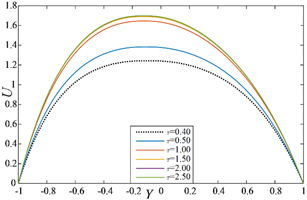Figure 4. Examine time sensibility on U

## 5.png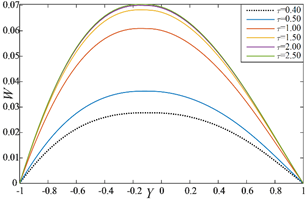Figure 5. Examine time sensibility on W

## 6.pngFigure 6. Examine time sensibility on $\theta$

6.3 Comparison

The accuracy of this study has been obtained by the following qualitative comparisons with the published results . By setting the values of some parameters, as mentioned in Figure 8, the present study (see Figure 8) coincides the published research. Figures 7 and 8 show that both the study are qualitatively also quantitatively quite same, which proofs the exactness of the current research.

## 7.png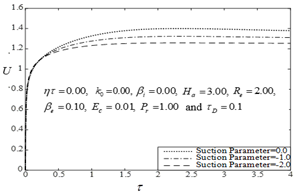Figure 7. Effect of S on U at U-plate

## 8.png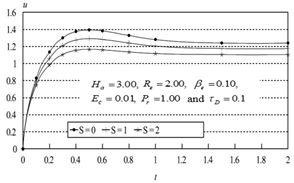Figure 8. Effect of S on U at U-plate (see )

6.4 Effect of parameters

To understand the inner physical situation of the flow model of the study, the impressions of some major parameters namely, Ion-slip parameter $\left( {{\beta }_{i}} \right)$ where ${{\alpha }_{e}}=1+{{\beta }_{i}}{{\beta }_{e}}$, Hartmann number $\left( {{H}_{a}} \right)$, phase angle$\left( \eta \tau \right)$ and Suction parameter $\left( S \right)$ in the appearance of Permeability parameter $\left( {{k}_{0}}=0.50 \right)$, Hall parameter $\left( {{\beta }_{e}}=0.10 \right)$, Reynolds number$\left( {{R}_{e}}=\text{3}.0 \right)$, Prandtl number $\left( {{P}_{r}}=0.8 \right),$ Eckert number $\left( {{E}_{c}}=0.01 \right)$ and Bingham number $\left( {{\tau }_{D}}=0.10 \right)$ at steady-state time $\tau =2.00$ are represented graphically through Figures 9-22.

The repercussion of both the primary and secondary velocities, temperature distributions, local shear stress as well as local Nusselt number against the impact of Hartmann number $\left( {{H}_{a}} \right)$ and Ion-slip parameter $\left( {{\beta }_{i}} \right)$ are represented in Figures 9-15. From Figures 9-11, it is checked that, primary velocity $U$decreases with the raise of $\left( {{H}_{a}} \right)$ while $W$ and $\theta$ both increases with the raise of $\left( {{H}_{a}} \right)$. Furthermore, $U$accelerates with the raise of $\left( {{\beta }_{i}} \right)$ whereas $W$ and $\theta$ both oppose with the raising values of $\left( {{\beta }_{i}} \right)$. From Figures 12-15, it is mentioned that the increasing values of $\left( {{H}_{a}} \right)$ opposes local shear stress on contrary growth of $\left( {{H}_{a}} \right)$ causes gradual increment in Nusselt number at both the plates. Furthermore, improvement in the local shear stress is marked with the enhancement of $\left( {{\beta }_{i}} \right)$ elsewhere, the Nusselt number monotonically declines when $\left( {{\beta }_{i}} \right)$ raises at both stationary and oscillatory plates.

## 9.pngFigure 9. Effects of ${{H}_{a}}$ and ${{\beta }_{i}}$ on $U$

## 10.pngFigure 10. Effects of ${{H}_{a}}$ and ${{\beta }_{i}}$ on $W$

## 11.png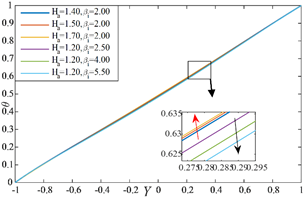Figure 11. Effects of ${{H}_{a}}$ and ${{\beta }_{i}}$ on $\theta$

## 12.png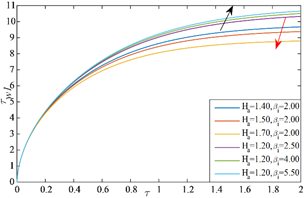Figure 12. Impacts of ${{H}_{a}}$ and ${{\beta }_{i}}$ on ${{\tau }_{w1}}$

## 13.png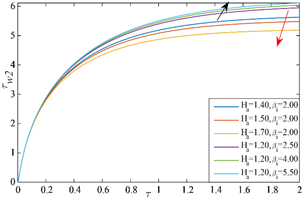Figure 13. Impacts of ${{H}_{a}}$ and ${{\beta }_{i}}$ on ${{\tau }_{w2}}$

## 14.png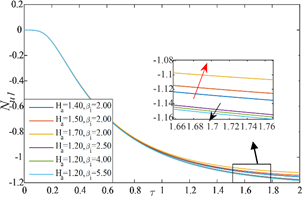Figure 14. Impacts of ${{H}_{a}}$ and ${{\beta }_{i}}$ on ${{N}_{u1}}$

## 15.png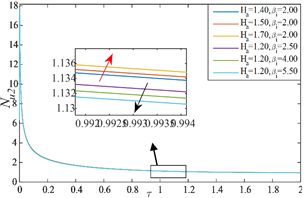Figure 15. Impacts of ${{H}_{a}}$ and ${{\beta }_{i}}$ on ${{N}_{u2}}$

## 16.png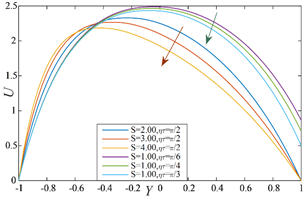Figure 16. Impressions of $S$ and $\eta \tau$on $U$

## 17.png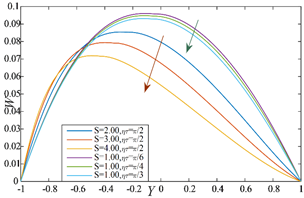Figure 17. Impressions of $S$ and $\eta \tau$on $W$

## 18.pngFigure 18. Impressions of $S$ and $\eta \tau$on $\theta$

## 19.png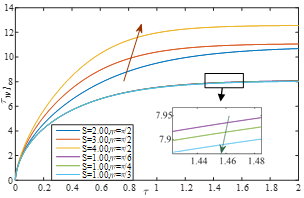Figure 19. Impressions of $S$ and $\eta \tau$ on ${{\tau }_{w1}}$

## 20.png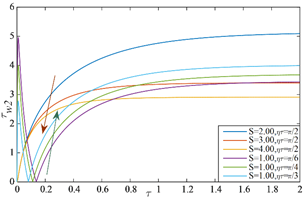Figure 20. Impressions of $S$ and $\eta \tau$ on ${{\tau }_{w2}}$

## 21.png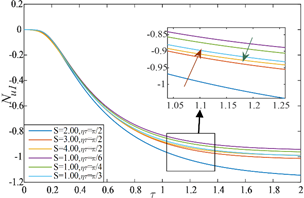Figure 21. Impressions of $S$ and $\eta \tau$on ${{N}_{u1}}$

## 22.png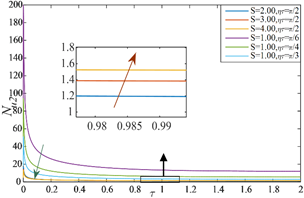Figure 22. Impressions of $S$ and $\eta \tau$on ${{N}_{u2}}$

Furthermore, the response of both the primary and secondary velocities, temperature distributions, local shear stress as well as local Nusselt number against the impression of Suction parameter $\left( S \right)$ and phase angle $\left( \eta \tau \right)$ have been represented in Figures 16-22. From Figures 16-18, it is detected that, all the profiles $U$, $W$ and $\theta$ decreases with the increment of $\left( S \right)$ and $\left( \eta \tau \right)$ both. It is observed from Figures 19-22, that the local shear stress raises subject to the increase of $\left( S \right)$ at the stationary plate while it decreases subject to the raise of $\left( S \right)$ at oscillatory plate. Elsewhere, the Nusselt number improves subject to the raise of $\left( S \right)$ at both stationary and oscillatory plates. Furthermore, a decreasing response on the local shear stress at the stationary plate is checked when the values of $\left( \eta \tau \right)$ develops whereas it increases at oscillatory plate with $\left( \eta \tau \right)$. On the other hand, at both the plates, the Nusselt number opposes when the values of $\left( \eta \tau \right)$ raises.

7. Conclusions

A numerical study is presented to describe the non-Newtonian flow behavior of the Bingham fluid with magnetohydrodynamic effects. Bingham fluid is considered flowing between two horizontally placed parallel plates where U-plate is in oscillation and L-plate is in a static position. The Finite difference technique is used as a solution algorithm. The discussions of results are kept limited for some significant parameters like as, Ion-slip parameter $\left( {{\beta }_{i}} \right)$, Hartmann number $\left( {{H}_{a}} \right)$, phase angle$\left( \eta \tau \right)$ and Suction parameter $\left( S \right)$. To make the study concise, the impacts of Permeability parameter $\left( {{k}_{0}} \right)$, Hall parameter $\left( {{\beta }_{e}} \right)$, Reynolds number$\left( {{R}_{e}} \right)$, Bingham number $\left( {{\tau }_{D}} \right)$, Prandtl number $\left( {{P}_{r}} \right)$ and Eckert number $\left( {{E}_{c}} \right)$ have not been shown. The salient outcomes of this study are given to the following:

1. The primary velocity is found to be increased with the raise of $\left( {{\beta }_{i}} \right)$ on contrary it decreases when $\left( {{H}_{a}} \right)$, $\left( \eta \tau \right)$ and $\left( S \right)$ increase.
2. The secondary velocity profile responses increasingly with the raise of $\left( {{H}_{a}} \right)$ while it decreases with the raise of $\left( {{\beta }_{i}} \right)$, $\left( \eta \tau \right)$ and $\left( S \right)$.
3. The temperature increases with the enlargement of $\left( {{H}_{a}} \right)$ while it decreases with the enlargement of $\left( {{\beta }_{i}} \right)$,$\left( \eta \tau \right)$ and $\left( S \right)$.
4. At the L-plate (stationary one), the rise of local shear stress happens with the improvement of $\left( {{\beta }_{i}} \right)$and $\left( S \right)$ while the improvement of $\left( \eta \tau \right)$ and $\left( {{H}_{a}} \right)$ pull it down.
5. At the U-plate (oscillatory one), the rise of local shear stress happens with the improvement of $\left( {{\beta }_{i}} \right)$and $\left( \eta \tau \right)$ while the improvement of $\left( S \right)$ and $\left( {{H}_{a}} \right)$ pull it down.
6. At both the plate, the local Nusselt number raises with the raise of $\left( {{H}_{a}} \right)$ and $\left( S \right)$ while increment on $\left( {{\beta }_{i}} \right)$and $\left( \eta \tau \right)$ cause fall on it.
Nomenclature
 $\tilde{u},\tilde{w}$ primary and secondary velocity components ${{T}_{1}},\text{ }{{T}_{2}}$ temperatures at lower and upper plates $\rho$ density of the ﬂuid $p$ Pressure of the fluid ${{B}_{0}}$ Uniform magnetic field $\tilde{\mu }$ Viscosity $\sigma$ electric conductivity of the fluid $k'$ magnetic permeability $\kappa$ thermal conductivity ${{c}_{p}}$ speciﬁc heat at the constant pressure $\omega$ frequency of the plate oscillation $U,W$ dimensionless Primary and secondary velocity components $\theta$ dimensionless temperature $\tau$ dimensionless time ${{\tau }_{w1}}$ dimensionless local shear stress at stationary plate ${{\tau }_{w2}}$ dimensionless local shear stress at oscillatory plate ${{N}_{u1}}$ dimensionless local Nusselt number at stationary plate ${{N}_{u2}}$ dimensionless local Nusselt number at oscillatory plate ${{T}_{m}}$ non-dimensional mean fluid temperature ${{\tau }_{D}}$ Bingham number or dimensionless yield stress ${{\beta }_{e}}$ Hall parameter ${{\beta }_{i}}$ Ion-slip parameter ${{R}_{e}}$ Reynolds number ${{P}_{r}}$ Prandtl number ${{E}_{C}}$ Eckert number $Ha$ Hartmann number $S$ suction Parameter ${{k}_{0}}$ permeability parameter $\eta \tau$ phase angle
References

 Stokes, G.G. (2010). On the effect of the internal friction of fluids on the motion of pendulums. In Mathematical and Physical Papers (Cambridge Library Collection - Mathematics,), pp. 1-10. Cambridge: Cambridge University Press. https://doi.org/10.1017/CBO9780511702266.002

 Datta, N., Jana, R.N. (1975). Oscillatory magetohydrodynamic flow past a flat plate with hall effects. Journal of Physical Society of Japan, 40(5): 1769-1474. https://doi.org/10.1143/JPSJ.40.1469

 El-Hakiem, M.A. (2000). MHD oscillatory flow on free convection-radiation through a porous medium with constant suction velocity. Journal of Magnetism and Magnetic Materials, 220(2-3): 271-276. https://doi.org/10.1016/S0304-8853(00)00444-3

 Chaudhary, R.C., Jain, A. (2007). Combined heat and mass transfer effects on MHD free convection flow past an oscillating plate embedded in porous medium. Romanian Journal of Physics, 52(5-7): 505-524.

 Bég, O.A., Zueco, J., Takhar, H.S. (2009). Unsteady magnetohydrodynamic Hartmann–Couette flow and heat transfer in a Darcian channel with Hall current, ionslip, viscous and Joule heating effects: Network numerical solutions. Communications in Nonlinear Science and Numerical Simulation, 14(4): 1082-1097. https://doi.org/10.1016/j.cnsns.2008.03.015

 Khan, I., Fakhar, K., Shafie, S. (2011). Magnetohydrodynamic free convection flow past an oscillating plate embedded in a porous medium. Journal of the Physical Society of Japan, 80(10): 104401-10. https://doi.org/10.1143/JPSJ.80.104401

 Rao, A., Raju, S., Sivaiah, S. (2012). Finite element solution of heat and mass transfer in MHD flow of a viscous fluid past a vertical plate under oscillatory suction velocity. Journal of Applied Fluid Mechanics, 5(3): 1-10. https://doi.org/10.36884/jafm.5.03.19435

 Manna, S.S., Das, S., Jana, R.N. (2012). Effects of radiation on unsteady MHD free convective flow past an oscillating vertical porous plate embedded in a porous medium with oscillatory heat flux. Advances in Applied Science Research, 3(6): 3722-3736.

 Ahmed, N., Kalita, H., Barua, D.P. (2012). An oscillatory three dimensional flow past an infinite vertical porous plate with soret and Dufour effects. International Journal of Heat and Technology, 30(2): 9-18. https://doi.org/10.18280/ijht.300202

 Hossain, M.D., Samad, M.A., Alam, M.M. (2015). MHD free convection and mass transfer flow through a vertical oscillatory porous plate with Hall, ion-slip currents and heat source in a rotating system. Procedia Engineering, 105(1): 56-63. https://doi.org/10.1016/j.proeng.2015.05.006

 Khalid, A., Khan, I., Khan, A., Shafie, S. (2015). Unsteady MHD free convection flow of Casson fluid past over an oscillating vertical plate embedded in a porous medium. Engineering Science and Technology, an International Journal, 18(3): 309-317. https://doi.org/10.1016/j.jestch.2014.12.006

 Hossain, M.D., Samad, M., Alam, M.M. (2016). MHD free convection and mass transfer flow through a vertical oscillatory porous plate in a rotating porous medium with hall, ion-slip currents and heat source. AMSE JOURNALS –2016-Series: Modelling B, 85(1): 28-42.

 Zin, N.A.M., Khan, I., Shafie, S. (2016). The impact silver nanoparticles on MHD free convection flow of Jeffrey fluid over an oscillating vertical plate embedded in a porous medium. Journal of Molecular Liquids, 222: 138-150. https://doi.org/10.1016/j.molliq.2016.06.098

 Kataria, H.R., Patel, H.R. (2016). Radiation and chemical reaction effects on MHD Casson fluid flow past an oscillating vertical plate embedded in porous medium. Alexandria Engineering Journal, 55(1): 583-595. https://doi.org/10.1016/j.aej.2016.01.019

 Sharmin, F., Alam, M.M. (2017). MHD viscoelastic fluid flow along an infinite oscillating porous plate with heat source and thermal diffusion. Modelling, Measurement and Control B, 86(4): 808-829. https://doi.org/10.18280/mmc_b.860401

 Singh, J.K., Rohidas, P., Joshi, N., Begum, S.G. (2017). Influence of hall and ion-slip currents on unsteady MHD free convective flow of a rotating fluid past an oscillating vertical plate. International Journal of Heat and Technology, 35(1): 37-52. https://doi.org/10.18280/ijht.350106

 Rangasamy, P., Murugesan, N. (2018). Soret and hall effect on unsteady free convection flow past an infinite vertical plate with oscillatory suction velocity and variable permeability. International Journal of Heat and Technology, 36(3): 808-816. https://doi.org/10.18280/ijht.360305

 Mollah, M.T., Islam, M.M., Alam, M.M. (2018). Couette Flow of Bingham Fluid with Ion-Slip and Hall Current. Lambert Academic Publishing, ISBN: 978-613-9-92592-6.

 Mollah, M.T., Islam, M.M., Alam, M.M. (2018). Hall and ion-slip effects on unsteady MHD Bingham fluid flow with suction. Modelling, Measurement and Control B, 87(4): 221-229. https://doi.org/10.18280/mmc_b.870402

 Mollah, M.T. (2019). EMHD laminar flow of Bingham fluid between two parallel Riga plates. International Journal of Heat and Technology, 37(2): 641-648. https://doi.org/10.18280/ijht.370236

 Khatun, S., Islam, M.M., Mollah, M.T., Poddar, S., Alam, M.M. (2021). EMHD radiating fluid flow along a vertical Riga plate with suction in a rotating system. SN Applied Sciences, 3(4): 1-14. https://doi.org/10.1007/s42452-021-04444-4

 Khatun, S., Mollah, M.T., Akter M.S., Islam, M.M., Alam, M.M. (2019). EMHD Couette flow of Bingham fluid through a porous parallel Riga plates with thermal radiation. Modelling, Measurement and Control B, 88(2-4): 106-113. https://doi.org/10.18280/mmc_b.882-409

 Attia, H.A., Sayed-Ahmedm, M.E. (2004). Hall effect on unsteady MHD Couette flow and heat of a Bingham fluid with suction and injection. Applied Mathematical Modeling, 28(12): 1027-1045. https://doi.org/10.1016/j.apm.2004.03.008

 Mollah, M.T., Poddar, S., Islam, M.M., Alam, M.M. (2021). Non-isothermal Bingham fluid flow between two horizontal parallel plates with ion-slip and hall currents. SN Applied Sciences, 3(1): 1-14. https://doi.org/10.1007/s42452-020-04012-2

 Mollah, M.T., Islam, M.M., Khatun, S., Alam, M.M. (2019). MHD generalized Couette flow and heat transfer on Bingham fluid through porous parallel plates. Mathematical Modelling of Engineering Problems, 6(4): 483-490. https://doi.org/10.18280/mmep.060402

 Akter, M.S., Islam, M.R., Mollah, M.T., Alam, M.M. (2019). Hall effects on Casson fluid flow along a vertical plate. In AIP Conference Proceedings, 2121(1): 040004. https://doi.org/10.1063/1.5115875

 Mollah, M.T., Islam, M.M., Ferdows, M., Alam, M.M. (2019). Bingham fluid flow through oscillatory porous plate with ion-slip and hall current. AIP Conference Proceedings, 2121(1): 050011. https://doi.org/10.1063/1.5115898

 Islam, M.M., Khatun, S., Mollah, M.T., Alam, M.M. (2019). Fluid flow along the Riga plate with the influence of magnetic force in a rotating system. AIP Conference Proceedings, 2121(1): 050002. https://doi.org/10.1063/1.5115889

 Islam, M.M., Mollah, M., Khatun, S., Ferdows, M., Alam, M.M. (2019). Unsteady viscous incompressible Bingham fluid flow through a parallel plate. Inventions, 4(3): 51. https://doi.org/10.3390/inventions4030051

 Islam, M.M., Mollah, M.T., Hasan, M.S., Alam, M.M. (2017). Numerical solution of unsteady viscous compressible fluid flow along a porous plate with induced magnetic field. Modelling, Measurement and Control B, 86(4): 850-863. https://doi.org/10.18280/mmc_b.860403

 Poddar, S., Islam, M.M., Ferdouse, J., Alam, M.M. (2021). Characteristical analysis of MHD heat and mass transfer dissipative and radiating fluid flow with magnetic field induction and suction. SN Applied Sciences, 3: 470. https://doi.org/10.1007/s42452-021-04452-4